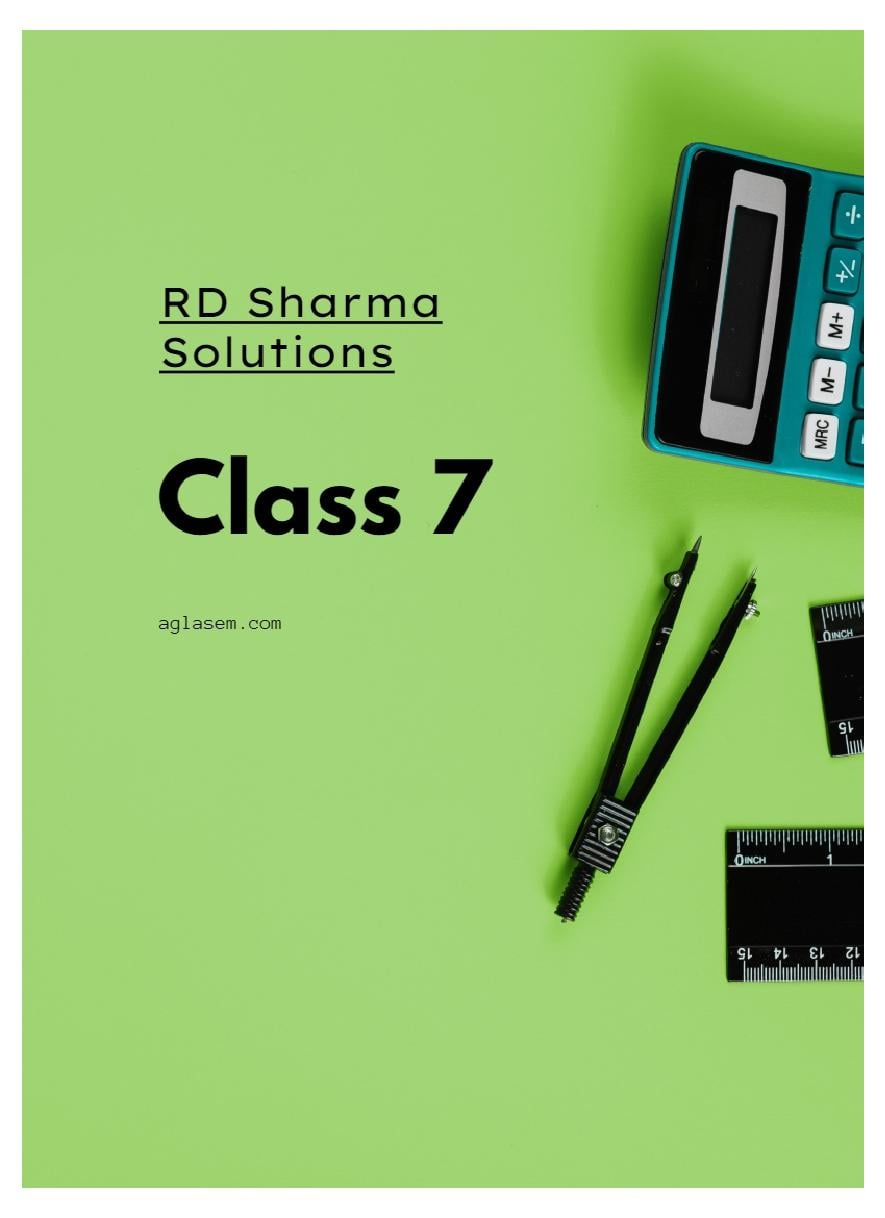aglasem.com

# RD Sharma Solutions Class 7 Chapter 8 Linear Equations in One Variable Exercise 8.3

Get here RD Sharma Solutions Class 7 Maths Chapter 8 Linear Equations in One Variable Exercise 8.3. All answers of Class 7 Maths RD Sharma Book for Chapter 8 Linear Equations in One Variable Exercise 8.3 are given in this PDF. More Detail